# REASONING (Test 1)

Tag: reasoning
Q.1
What comes next?A.B.C.D.Explaination / Solution:

The rectangle starting on the left is moving from left to right one place at a time at each stage.

Workspace
Report
Q.2
A person traveled a distance of 50 km in 8 hours. He covered a part of the distance on foot at the rate of 4 km per hour and a part on a bicycle at the rate of 10 km per hour. How much distance did he travel on foot?
A. 10 km
B. 20 km
C. 30 km
D. 40 km
Explaination / Solution:
No Explaination.

Workspace
Report
Q.3
In how many ways can four children be made to stand in a line such that two of them, A and B are always together?
A. 6
B. 12
C. 18
D. 24
Explaination / Solution:
No Explaination.

Workspace
Report
Q.4
As per agreement with a bank, a businessman had to refund a loan in some equal installments without interest. After paying 18 installments he found that 60 percent of his loan was refunded. How many installments were there in the agreement?
A. 22
B. 24
C. 30
D. 33
Explaination / Solution:
No Explaination.

Workspace
Report
Q.5
Four children are sitting in a row. A is occupying the seat next to B but not next to C. If C is not sitting next to D, who is/are occupying seat/seats adjacent to D?
A. B
B. A
C. B and A
D. Impossible to tell
Explaination / Solution:
No Explaination.

Workspace
Report
Q.6
Find the number of triangles in the given figure.A. 8
B. 10
C. 12
D. 14
Explaination / Solution:

The figure may be labelled as shown.The simplest triangles are AHG, AIG, AIB, JFE, CJE and CED i.e. 6 in number.

The triangles composed of two components each are ABG, CFE, ACJ and EGI i.e. 4 in number.

The triangles composed of three components each are ACE, AGE and CFD i.e. 3 in number.

There is only one triangle i.e. AHE composed of four components.

Therefore, There are 6 + 4 + 3 + 1 = 14 triangles in the given figure.

Workspace
Report
Q.7
Find the number of triangles in the given figure.A. 21
B. 23
C. 25
D. 27
Explaination / Solution:

The figure may be labelled as shown.The simplest triangles are ABL, BCD, DEF, FGP, PGH, QHI, JQI, KRJ and LRK i.e. 9 in number.

The triangles composed of two components each are OSG, SGQ, SPI, SRI, KSQ, KMS, FGH, JHI and JKL i.e. 9 in number.

There is only one triangle i.e. KSG which is composed of four components.

The triangles composed of five components each are NEI, ANI, MCG and KCO i.e. 4 in number.

The triangles composed of six components each are GMK and KOG i.e. 2 in number.

There is only one triangle i.e. AEI composed of ten components.

There is only one triangle i.e. KCG composed of eleven components.

Therefore, Total number of triangles in the given figure = 9 + 9+1 + 4 + 2+1 + 1 = 27.

Workspace
Report
Q.8

Find the number of triangles in the given figure.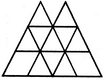A. 16
B. 18
C. 14
D. 15
Explaination / Solution:

The figure may be labelled as shown.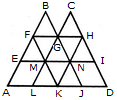The simplest triangles are BFG, CGH, EFM, FMG, GMN, GHN, HNI, LMK, MNK and KNJ i.e. 10 in number.

The triangles composed of three components each are FAK and HKD i.e. 2 in number.

The triangles composed of four components each are BEN, CMI, GLJ and FHK i.e. 4 in number.

The triangles composed of eight components each are BAJ and OLD i.e. 2 in number.

Thus, there are 10 + 2 + 4 + 2 = 18 triangles in the given figure.

Workspace
Report
Q.9
Find the number of triangles in the given figure.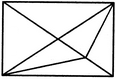A. 11
B. 13
C. 15
D. 17
Explaination / Solution:

The figure may be labelled as shown.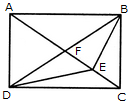The simplest triangles are AFB, FEB, EBC, DEC, DFE and AFD i.e. 6 in number.

The triangles composed of two components each are AEB, FBC, DFC, ADE, DBE and ABD i.e. 6 in number.

The triangles composed of three components each are ADC and ABC i.e. 2 in number.

There is only one triangle i.e. DBC which is composed of four components.

Thus, there are 6 + 6 + 2 + 1 = 15 triangles in the figure.

Workspace
Report
Q.10
In the adjoining figure, if the centres of all the circles are joined by horizontal and vertical lines, then find the number of squares that can be formed.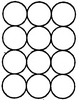A. 6
B. 7
C. 8
D. 1
Explaination / Solution:

The figure may be labelled as shown.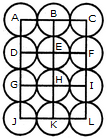We shall join the centres of all the circles by horizontal and vertical lines and then label the resulting figure as shown.

The simplest squares are ABED, BCFE, DEHG, EFIH, GHKJ and HILK i.e. 6 in number.

The squares composed of four simple squares are ACIG and DFLJ i.e. 2 in number.

Thus, 6 + 2 = 8 squares will be formed.

Workspace
Report